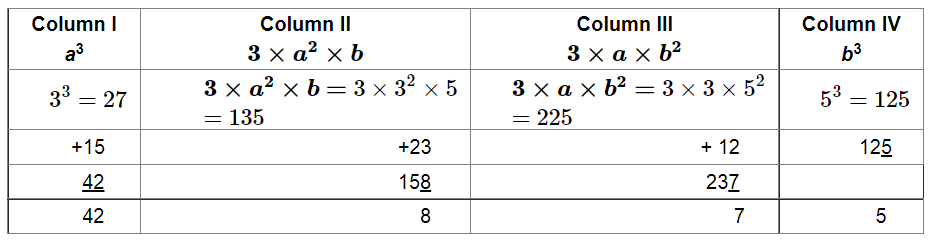# Find the cubes of the following numbers by column method:`
Question:

Find the cubes of the following numbers by column method:

(i) 35

(ii) 56

(iii) 72

Solution:

(i)

We have to find the cube of 35 using column method. We have: $a=3$ and $b=5$